## Dividing Decimals by Whole Number

Practice Unlimited Questions

#### How to Divide a Decimal Number by a Whole Number?

Step 1: Divide the ones.

Step 2: Add the tenths then divide.

Step 3: Add the hundredths then divide.

See working examples below.

#### 1. Bella used 0.6 m cloth to make 3 similar handkerchiefs. How many metres of cloth did she use for each handkerchief?

Divide 0.6 m by 3.
0.6  ÷  3  =  ?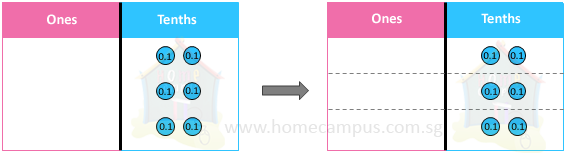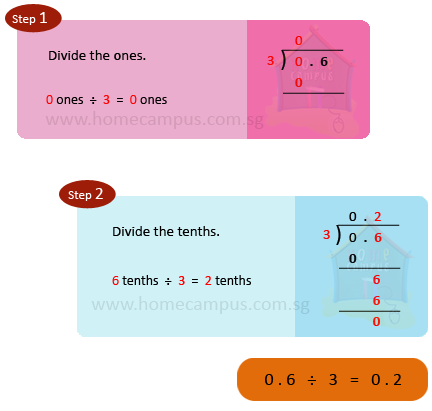Bella used 0.2 m of cloth for each handkerchief.

#### 2. Bella used 6 m cloth to make 4 similar scarves. How much cloth did she use for each scarf?

Divide 6 m by 4.
6  ÷  4  =  ?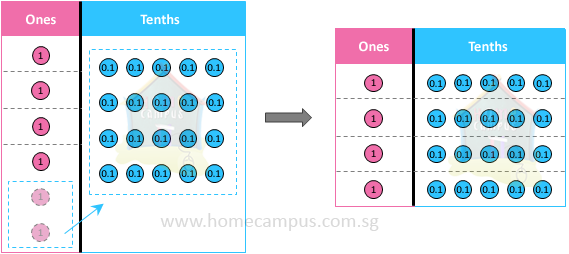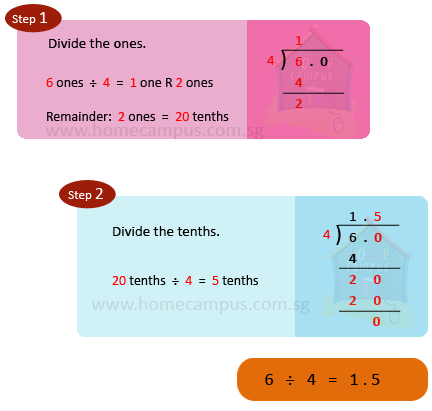Bella used 1.5 m cloth for each scarf.

#### 3. Bella had 7.25 m cloth that she used to make 5 bags of the same size. How many m of cloth did she use for each bag?

Divide 7.25 m by 5.
7.25  ÷  5  =  ?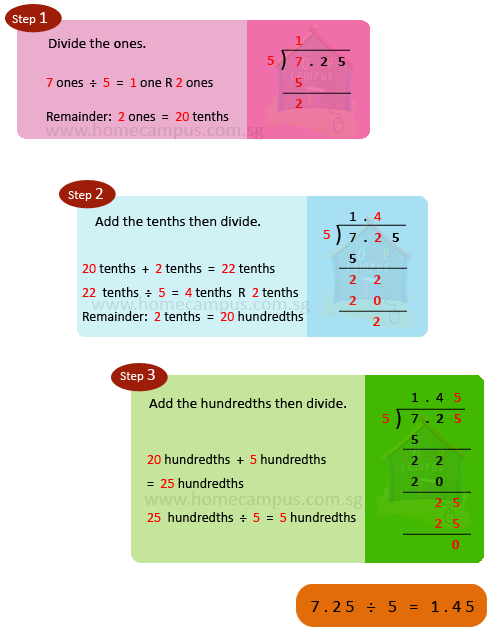Bella used 1.45 m of cloth for each bag.

#### 4. Serena bought 5 erasers for \$4 and 7 markers for \$15.75. a) Find the cost of each eraser. b) Find the cost of each marker.

a) Cost of 5 erasers   =   \$4
Cost of 1 eraser     =   \$4  ÷  5
=   \$0.80

The cost of each eraser is \$0.80.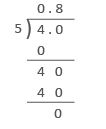b) Cost of 7 markers   =   \$15.75
Cost of 1 marker     =   \$15.75  ÷  7
=   \$2.25

The cost of each marker is \$2.25.